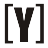# College GPA Calculator

 Class Name CreditHours Grade - select - A A- B+ B B- C+ C C- D+ D D- F P NP GPA:

## How to calculate GPA

GPA is equal to the sum of the credit hours weight (w) multiplied by grade (g)

FORMULA:

`GPA = w1×g1+ w2×g2+ w3×g3 + ... + wn×gn`

credit hours weight (wi):

`wi= ci / (c1+c2+c3+...+cn)`

A 4.00
A- 3.67
B+ 3.33
B 3.00
B- 2.67
C+ 2.33
C 2.00
C- 1.67
D+ 1.33
D 1.00
D- 0.67
F 0
P (pass)
NP (no pass)YoosFuhl.com Would you like to receive notifications on latest updates? No Yes